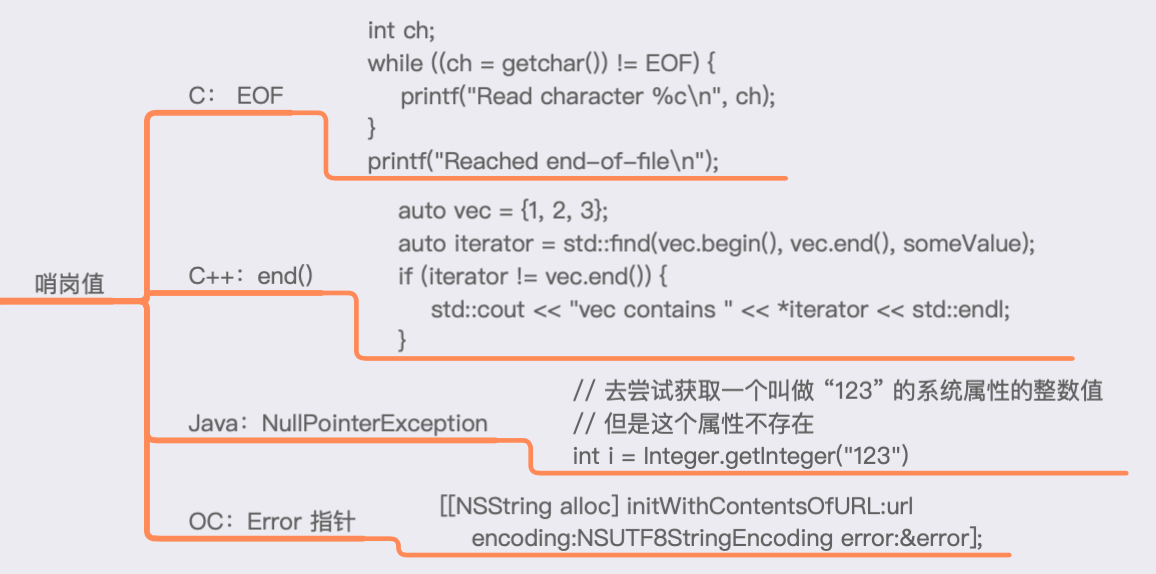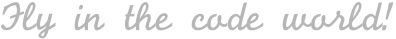| Swift , 阅读笔记

### 内容概览

• 1.哨岗值
• 2.通过枚举解决魔法数的问题
• 3.可选值概览
• 4.强制解包的时机
• 5.隐式解包可选值

## 1. 哨岗值## 2. 通过枚举解决魔法数的问题

``````enum Optional<Wrapped> {
case none
case some(Wrapped)
}
``````

``````var array = ["one","two","three"]
switch array.firstIndex(of: "four") {
case .some(let idx):
array.remove(at: idx)
case .none:
break // 什么都不做
}
``````

``````var array = ["one","two","three"]
switch array.firstIndex(of: "four") {
case let idx?:
array.remove(at: idx)
case nil:
break // 什么都不做
}
``````

## 3. 可选值概览

### if let

``````var array = ["one", "two", "three", "four"]
// 可以把布尔限定语句与 if let 搭配在一起使用
if let idx = array.firstIndex(of: "four"), idx != array.startIndex {
// idx 只在这个 if let 语句的作用域中有效
array.remove(at: idx)
}
``````

``````if let url = URL(string: urlString),
url.pathExtension == "png", // 多个 let 的任意部分也能拥有布尔值限定的语句
let data = try? Data(contentsOf: url), // 通过 try? 来转变为一个可选值
let image = UIImage(data: data) {
let view = UIImageView(image: image)
}
``````

### while let

``````// 可以在可选绑定后面添加一个布尔值语句
while let line = readLine(), !line.isEmpty {
print(line)
}
``````

``````let array = [1, 2, 3]
// 创建迭代器
var iterator = array.makeIterator()

// 迭代器中的 next 方法将不断返回序列中的值，
// 并在序列中的值被耗尽的时候，返回 nil
while let i = iterator.next() {
print(i, terminator: " ")
}
``````

`for` 循环也支持布尔语句，只是要在布尔语句之前，使用 `where` 关键字：

``````for i in 0..<10 where i % 2 == 0 {
print(i, terminator: " ")
}
``````

``````var iterator2 = (0..<10).makeIterator()
while let i = iterator2.next() {
guard i % 2 == 0 else { continue }
print(i)
}
``````

### 双重可选值

``````let stringNumbers = ["1", "2", "three"]
let maybeInts = stringNumbers.map { Int(\$0) }

for maybeInt in maybeInts {
// maybeInt 是一个 Int? 值
// 得到两个整数值和一个 `nil`
}

// for...in 是 while 循环加上一个迭代器的简写方式
var iterator = maybeInts.makeIterator()
while let maybeInt = iterator.next() {
print(maybeInt, terminator: " ") // 得到两个整数值和一个 `nil`
}
``````

``````for case let i? in maybeInts { // 或者 for case let .some(i) in maybeInts
// i 将是 Int 值，而不是 Int?
print(i, terminator: " ")
}
``````

`x?` 这个模式，它只会匹配那些非 `nil` 的值，是 `.some(x)` 的简写形式。

``````for case nil in maybeInts {
// 将对每个 nil 执行一次
print("No value")
}
``````

``````let j = 5
if case 0..<10 = j {
print("\(j) 在范围内")
} // 5 在范围内
``````

### if var and while var

``````let number = "1"
if var i = Int(number) {
i += 1
print(i)
}
``````

### 解包后可选值的作用域

``````extension String {
var fileExtension: String? {
let period: String.Index
if let idx = lastIndex(of: ".") {
period = idx
} else {
return nil
}
let extensionStart = index(after: period)
return String(self[extensionStart...])
}
}
``````

``````extension String {
var fileExtension: String? {
guard let period = lastIndex(of: ".") else {
return nil
}
let extensionStart = index(after: period)
return String(self[extensionStart...])
}
}
``````

guard - else

• guard 能够接受任何在普通的 if 语句中能接受的条件。
• 条件不成立时，提前退出。

• 可以有效地减少因大量使用 `if - else` 而导致的代码块缩进，使代码更优雅、易读；
• 可以有效地提升防御式编程思维，督促开发者多思考 `条件不成立` 的情况；

Swift 中的“无”类型

• “东西不存在”(`nil`)
• “存在且为空”(`Void`), `public typealias Void = ()`
• 常用做那些不返回任何东西的函数的返回值。
• “不可能发生” (`Never`), `public enum Never { }`
• 一个返回 Never 的函数用于通知编译器:它绝对不会返回。一种是像 fatalError 那样表示程序失败的函数，另一种是像 dispatchMain 那样 运行在整个程序生命周期的函数。

### 可选链

delegate?.callback()，加上 `?` 来表示你正在链接这个可选值。

``````var a: Int? = 5
a? = 10
a // Optional(10)

var b: Int? = nil
b? = 10
b // nil
``````

### nil 合并运算符 ??

``````let stringteger = "1"
let number = Int(stringteger) ?? 0
``````

``````let i: Int? = nil
let j: Int? = nil
let k: Int? = 42
i ?? j ?? k ?? 0 // 按顺序合并，最终得到 42
``````

``````let s1: String?? = nil // nil
(s1 ?? "inner") ?? "outer" // inner
let s2: String?? = .some(nil) // Optional(nil)
(s2 ?? "inner") ?? "outer" // outer
``````

### 在字符串插值中使用可选值

``````let bodyTemperature: Double? = 37.0
let bloodGlucose: Double? = nil
print(bodyTemperature) // Optional(37.0)
``````

• 显式地用 as Any 进行转换，使用 ! 对值进行强制解包 (如果你能确定该值不为 nil 时)
• 使用 String(describing: …) 对它进行包装
• 用 nil 合并运算符提供一个默认值

``````infix operator ???: NilCoalescingPrecedence
public func ???<T>(optional: T?, defaultValue: @autoclosure () -> String)
-> String {
switch optional {
case let value?: return String(describing: value)
case nil: return defaultValue()
}
}

print("Body temperature: \(bodyTemperature ??? "n/a")")
// Body temperature: 37.0
``````

### 可选值 map

``````var firstCharAsString: String? = nil
if let char = characters.first {
firstCharAsString = String(char)
}
``````

``````let firstChar = characters.first.map { String(\$0) } // Optional("a")
``````

`map` 的实现代码：

``````extension Optional {
func map<U>(transform: (Wrapped) -> U) -> U? {
guard let value = self else { return nil }
return transform(value)
}
}
``````

### 可选值 flatMap

`flatMap` 可以把结果展平为单个可选值，避免多重嵌套的可选值（如：`Int??`）。

``````let urlString = "https://www.objc.io/logo.png"
if let url = URL(string: urlString),
let data = try? Data(contentsOf: url),
let image = UIImage(data: data) {
let view = UIImageView(image: image)
}
``````

`flatMap` 进行等价转换：

``````let urlString = "https://www.objc.io/logo.png"
let view = URL(string: urlString)
.flatMap { try? Data(contentsOf: \$0) }
.flatMap { UIImage(data: \$0) }
.map { UIImageView(image: \$0) }
``````

`flatMap` 的实现代码：

``````extension Optional {
func flatMap<U>(transform: (Wrapped) -> U?) -> U? {
if let value = self, let transformed = transform(value) {
return transformed
}
return nil
}
}
``````

### 使用 compactMap 过滤 nil

``````let numbers = ["1", "2", "3", "foo"]
var sum = 0
for case let i? in numbers.map({ Int(\$0) }) {
sum+=i
}
sum // 6
``````

``````numbers.map { Int(\$0) }.reduce(0) { \$0 + (\$1 ?? 0) } // 6
``````

``````numbers.compactMap { Int(\$0) }.reduce(0, +) // 6
``````

``````extension Sequence {
func compactMap<B>(_ transform: (Element) -> B?) -> [B] {
// 使用 lazy 可以避免多个作为中间结果的数组的内存分配
return lazy.map(transform).filter { \$0 != nil }.map { \$0! }
}
}
``````

### 可选值判等

• 两者都是 `nil`
• 两者都有值；
• 两者中有一个有值， 另一个是 `nil`

``````extension Optional: Equatable where Wrapped: Equatable {
static func ==(lhs: Wrapped?, rhs: Wrapped?) -> Bool {
switch (lhs, rhs) {
case (nil, nil): return true
case let (x?, y?): return x == y
case (_?, nil), (nil, _?): return false
}
}
}
``````

``````dictWithNils["two"] = Optional(nil)
dictWithNils["two"] = .some(nil)
``````

``````dictWithNils["two"]? = nil
``````

## 4. 强制解包的时机

### 改进强制解包的错误信息

``````infix operator !!
func !! <T>(wrapped: T?, failureText: @autoclosure () -> String) -> T {
if let x = wrapped { return x }
fatalError(failureText())
}

let s = "foo"
let i = Int(s) !! "Expecting integer, got \"\(s)\""
``````

### 在调试版本中进行断言

``````infix operator !?
func !? <T: ExpressibleByIntegerLiteral>(wrapped: T?, failureText: @autoclosure () -> String) -> T {
assert(wrapped != nil, failureText())
return wrapped ?? 0
}

let s = "20"
let i = Int(s) !? "Expecting integer, got \"\(s)\""
``````

``````func !?<T>(wrapped: T?, nilDefault: @autoclosure () -> (value: T, text: String)) -> T {
assert(wrapped != nil, nilDefault().text)
return wrapped ?? nilDefault().value
}
// 调试版本中断言，发布版本中返回 5 Int(s) !? (5, "Expected integer")
``````

• fatalError，接受一条信息，并且 `无条件地停止` 操作。
• 使用 assert 来检查条件，当条件结果为 false 时，停止执行并输出信息。`在发布版本中，assert会被移除掉`，条件不会被检测，操作也永远不会挂起。
• 使用 precondition，它和 assert 有一样的接口，但是`在发布版本中不会被移除`。只要条件被判定为 false，执行就会被停止。

## 5. 隐式解包可选值

• Objective-C 中表示引用是否 可以为空的语法是最近才被引入的，以前除了假设返回的引用可能是 nil 引用以外，也没有什么好办法。
• 所有人都已经习惯了 Objective-C 世界中对象 “可能为空” 的设定，因此把这样的返回值作为隐式解包可选值来使用是可以说得过去的。

• 最常见的情况就是两阶段初始化 (two-phase initialization)。当你的类准备好被使用时，所有的隐式解包可选值都将有一个值。这就是 Xcode 和 Interface Builder 在 view controller 的生命周期中使用它们的方式。

#### 隐式可选值行为

``````var s: String! = "Hello"
s?.isEmpty // Optional(false)
if let s = s { print(s) } // Hello
s = nil
s ?? "Goodbye" // Goodbye
``````

#### 评论区(期待你的留言)